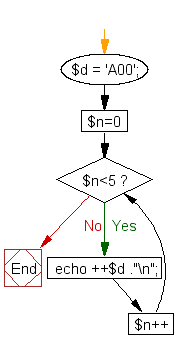﻿ PHP Exercise: Using a character type variable print numbers - w3resource

# PHP Exercises : Using a character type variable print numbers

## PHP : Exercise-19 with Solution

Arithmetic operations on character variables : \$d = 'A00'. Using this variable print the following numbers.

Sample Solution: -

PHP Code:

``````<?php
\$d = 'A00';
for (\$n=0; \$n<5; \$n++)
{
echo ++\$d ."\n";
}
?>
```
```

Sample Output:

```A01
A02
A03
A04
A05
```

Flowchart:PHP Code Editor:

Have another way to solve this solution? Contribute your code (and comments) through Disqus.

What is the difficulty level of this exercise?

Test your Programming skills with w3resource's quiz.

﻿

## PHP: Tips of the Day

Returns all elements in an array except for the first one

Example:

```<?php
function tips_tail(\$items)
{
return count(\$items) > 1 ? array_slice(\$items, 1) : \$items;
}

print_r(tips_tail([1, 5, 7]));
?>
```

Output:

```Array
(
 => 5
 => 7
)
```